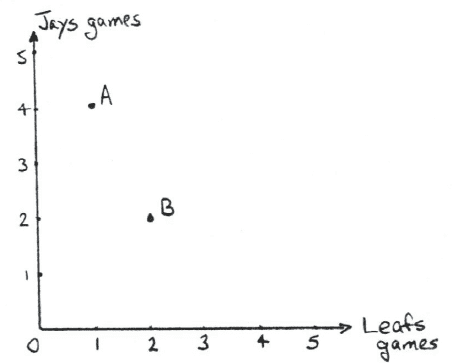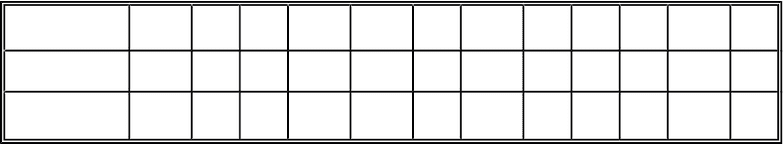Class Notes (1,100,000)
CA (650,000)
UTSC (30,000)
Lecture 1

# MGEB02H3 Lecture Notes - Lecture 1: Problem Set, Indifference Curve, List Of Sports Attendance Figures

Department
Economics for Management Studies
Course Code
MGEB02H3
Professor
A.Mazaheri
Lecture
1

This preview shows pages 1-3. to view the full 23 pages of the document.MGEB02F Problem Set 1 Professor Michael Krashinsky
1. A group of Toronto sports fans meet regularly to discuss their feelings about Toronto's hockey and
baseball teams (the "Leafs" and the "Blue Jays"). One day, the fans express how they feel about the
relative advantages of attending hockey and baseball games in Toronto. One of the fans - a past student in
ECOB02 - decides to draw indifference curves to represent the preferences of each of the fans in the
group (the fun never stops when economists are around!). Your mission is to draw these indifference
curves, using a separate diagram for each fan. In each case, you should draw two indifference curves, one
passing through point A (where the fan attends 1 Leaf games and 4 Blue Jay games) and the other passing
through point B (where the fan goes to 2 Leafs games and 2 Blue Jays Games). For each fan, compute
the MRS at points A and B. Also explain which indifference curves satisfy the common assumptions
about indifference curve and which do not (explain which assumptions are violated). In each case explain
how the indifference curves shift if prices change. Note: some of these indifference curves may look
strange, but that is OK (for one thing, you are reminded that the word "fan" comes from the word
"fanatic").
In each case you should draw two sets of indifference curves. In the first set, assume that fans can only
attend an integer number of games of either type (that is, the fan can attend 1 game or 2 games or 3 games
etc., but not 2.3 games or 4.7 games, etc.). In the second set, pretend that fans can attend fractional games
(so that you can attend 2.3 games or 4.7 games, etc.). Hint: the only difference is that the first set consists
of isolated points on the graph, while the second set allows you to connect the points to get continuous
lines).
a) Fan #1 values each game according to the length of the wooden object used to strike the ball
or puck. Hockey sticks are generally twice as long as bats.
b) Fan #2 values only baseball games and refuses to attend a hockey game until the NHL does
something about goons fighting each other.
c) Fan #3 like both sports equally, but, ever since the drug scandal in baseball, refuses to attend
more than four Blue Jays games in a season (hence any baseball games beyond four have no
value).

Only pages 1-3 are available for preview. Some parts have been intentionally blurred.d) Fan #4 values balance in sports attendance, and must attend one baseball game for every
hockey game. Any extra games in either sport are considered useless.
e) Fan #5 also values balance, but not in as extreme a way as fan #4. Fan #5 considers useless
more than two hockey games for every baseball game, or more than two baseball games for
every hockey game. In between, fan #5 considers the two games as interchangeable (that is, one
Leaf game is worth the same as one Jays game).
f) Fan #6 cannot mix sports. Each year this fan watches only hockey or baseball (because of a
prior agreement with this fan's spouse, watching two sports in one year is grounds for divorce).
Given a choice, the fan attempts to maximize the number of games attended, and does not care
which sport this is (that is, given tickets to both sports, the fan will choose the sport for which
there are the most tickets, and will discard the other tickets).
g) Fan #7 loves both sports equally, but cannot attend more than five sporting events in any one
year (in this case, there is also a prior agreement with this fan's spouse, and attending more than
five games in one year is grounds for divorce). Thus, tickets for more than five games total are
useless.
2. You are given the following twelve consumption bundles (A through L) representing the possible
weekly consumption of chicken and beef by a rather large family (the amount of chicken and beef is
given in kilograms):
Bundle A B C D E F G H I J K L
Beef 1 4 7 14 2 7 15 2 9 8 11 3
Chicken 13 6 3 1 14 5 2 7 2 8 2 8
You are told that the family's utility function for each of the first seven bundles is as follows:
U(A)=U(B)=U(C)=U(D)=7 and U(E)=U(F)=U(G)=13, and that this function satisfies the usual
assumptions, including convexity.
a) Indicate, for each of the remaining 5 bundles (H, I, J, K, and L) the possible values of the
utility function. That is, if you determined that bundle "X" was clearly preferred to bundles E,F,
and G, you could write U(X)>13. You will find a graph very helpful in solving this problem.
b) Do you know the exact value of the MRS at point C? If not, can you find some range within
which the MRS will lie?
c) Now suppose that you are given a 13th bundle with 10 Kg of beef and 2 Kg of chicken, and
you are told that the utility of this bundle is 8. How does that affect your answer to part b?

Only pages 1-3 are available for preview. Some parts have been intentionally blurred.

3. A consumer has the following utility function for two goods F (food) and H (housing): U =
(F-4)(H-3) (assuming F>4, H>3). Assume that the graph of the indifference curve is drawn
with H on the horizontal axis. We want to determine the MRS at the point H=13, F=7 (the
indifference curve through that point corresponds to U=30). We will do this several different
ways:
a) Draw a graph of the indifference curve and measure the MRS.
b) Starting at that point, increase H by 1 unit and determine the reduction in F necessary to keep
utility constant at 30. Explain why this is only an estimate of the MRS.
c) Obtain a better estimate by repeating b, increasing H by 0.01.
d) Obtain an explicit expression for the indifference curve (F as a function of H) and find the
derivative. Evaluate -dF/dH at the point.
e) Derive the implicit function for the indifference curve, totally differentiate and obtain -dF/dH,
and evaluate this at the point.
f) Compute the marginal utilities of food and housing at this point and express the MRS as ratio
of these marginal utilities.
g) We also want to illustrate the point that the MRS does not change when we take any
monotonically increasing transformation of the utility function. To do this, use the method part f
to obtain the MRS in the following cases:
i) we transform the utility function by adding 10
ii) we transform the utility function by squaring it
iii) we transform the utility function by adding 6 and cubing it
h) On a fresh piece of graph paper, draw the indifference curves for utility levels 18, 30 and 42.
Place H on the horizontal axis and F on the vertical axis. Run the H-axis from 0 to 35 and the F-
axis from 0 to 25. Now, on the same graph, draw the budget line for each of the following
combinations of income (I), the price of food (PF), and the price of housing (PH). Determine the
consumption bundle purchased by the consumer in each case:
i) I = 66, PF = 3, PH = 6
ii) I = 130, PF = 7, PH = 6
iii) I = 109, PF = 10, PH = 3
i) In each of the three cases in part h, solve the constrained maximization problem to show that
your graphical answer is correct. [The purpose of this problem is to "convince" you that
Lagrangians really work!]
4. It is important to understand intuitively what happens to the budget line when prices and/or income
change. To practice, we will begin with the budget line corresponding to I = 24, PX = 6, PY = 4. Now,
sketch this budget line and what happens to it in each of the following 8 cases:
a) PX doubles
b) PX is halved